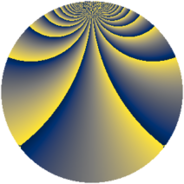# Properties

 Label 1849.4.oLevel $1849$ Weight $4$ Character orbit 1849.o Rep. character $\chi_{1849}(9,\cdot)$ Character field $\Q(\zeta_{903})$ Dimension $237888$ Sturm bound $630$

# Related objects

## Defining parameters

 Level: $$N$$ $$=$$ $$1849 = 43^{2}$$ Weight: $$k$$ $$=$$ $$4$$ Character orbit: $$[\chi]$$ $$=$$ 1849.o (of order $$903$$ and degree $$504$$) Character conductor: $$\operatorname{cond}(\chi)$$ $$=$$ $$1849$$ Character field: $$\Q(\zeta_{903})$$ Sturm bound: $$630$$

## Dimensions

The following table gives the dimensions of various subspaces of $$M_{4}(1849, [\chi])$$.

Total New Old
Modular forms 238896 238896 0
Cusp forms 237888 237888 0
Eisenstein series 1008 1008 0

## Trace form

 $$237888q - 504q^{2} - 507q^{3} + 3272q^{4} - 521q^{5} - 494q^{6} - 462q^{7} - 518q^{8} - 4875q^{9} + O(q^{10})$$ $$237888q - 504q^{2} - 507q^{3} + 3272q^{4} - 521q^{5} - 494q^{6} - 462q^{7} - 518q^{8} - 4875q^{9} - 475q^{10} - 468q^{11} - 630q^{12} - 345q^{13} + 2240q^{14} - 353q^{15} + 14612q^{16} - 592q^{17} - 444q^{18} + 3116q^{19} - 1137q^{20} - 709q^{21} + 4q^{22} - 3849q^{23} - 1588q^{24} - 11979q^{25} - 383q^{26} - 696q^{27} - 1744q^{28} - 499q^{29} - 4439q^{30} + 2967q^{31} + 2214q^{32} - 989q^{33} + 527q^{34} - 10939q^{35} + 99451q^{36} - 288q^{37} - 2372q^{38} - 1766q^{39} - 3189q^{40} - 120q^{41} - 5802q^{42} - 2305q^{43} + 4160q^{44} - 1372q^{45} - 4847q^{46} - 1726q^{47} - 5759q^{48} + 136576q^{49} + 2757q^{50} + 4287q^{51} + 3086q^{52} - 203q^{53} + 646q^{54} + 944q^{55} + 3447q^{56} - 958q^{57} - 658q^{58} - 1678q^{59} - 4069q^{60} - 1353q^{61} + 3443q^{62} - 3795q^{63} + 52902q^{64} - 570q^{65} + 3910q^{66} - 2058q^{67} - 7164q^{68} + 4199q^{69} + 2037q^{70} + 1103q^{71} - 62251q^{72} + 3114q^{73} + 4392q^{74} - 89544q^{75} - 4360q^{76} - 4031q^{77} - 3410q^{78} - 3378q^{79} + 1094q^{80} - 33849q^{81} + 35988q^{82} - 11120q^{83} - 16879q^{84} - 9182q^{85} - 2374q^{86} - 21002q^{87} - 7806q^{88} - 11994q^{89} - 131729q^{90} - 12436q^{91} - 1306q^{92} - 512q^{93} + 37440q^{94} - 3560q^{95} + 4662q^{96} + 57004q^{97} + 4738q^{98} - 7699q^{99} + O(q^{100})$$

## Decomposition of $$S_{4}^{\mathrm{new}}(1849, [\chi])$$ into newform subspaces

The newforms in this space have not yet been added to the LMFDB.